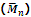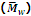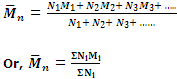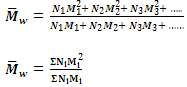Explain the molecular mass with respect to polymers.

During the formation of polymers, different macromolecules have different degree of polymerisation i.e. they have varied chain lengths. Thus, the molecular masses of the individual macromolecules in a particular sample of the polymer are different. Hence, an average value of the molecular mass is taken. There are two kinds of average molecular masses of polymers.

1. Number-average molecular mass2. Mass-average molecular massThe two types of molecular masses are defined and calculated as follows:

1. Number-average molecular mass

When the total mass of all the molecules of a sample is divided by the total number of molecules, the result obtained is called the number-average molecular mass. For example, suppose in a particular sample

N1 molecules have molecular mass M1 each.

N2 molecules have molecular mass M2 each.

N3 molecules have molecular mass M3 each and so on. Then, we have

Total mass of all the N1 molecules = N1M1.

Total mass of all the N2 molecules = N2M2.

Total mass of all the N3 molecules = N3M3 and so on.

Total mass of all the molecules = N1M1 + N2M2 + N3M3 + .....

= ΣNiMi

Total number of all the molecules = N1 + N2 + N3 + ....

= ΣNi

Hence the number-average molecular mass will be given byis generally determined by osmotic pressure measurement.

2. Mass-Average molecular mass

When the total mass of groups of molecules having different molecular masses are multiplied with their respective molecular masses, the products are added and the sum is divided by the total mass of all the molecules, the result obtained is called the mass-average molecular mass. Supposing, as before that N1N2N3, etc, molecules have molecular mass M1M2M3 etc. correspondingly.

Total mass of N1 molecules = N1M1.

Total mass of N2 molecules = N2M2.

Total mass of N3 molecules = N3M3 and so on.

The products with their respective molecular masses will be (N1M1 × M1)(N2M2 × M2)(N3M3 × M3), etc. i.e. N1M12N2M22N3M32, etc.

Sum of the products = N1M12 + N2M22 + N3M32 + ......

= ΣNiMi2

Hence the mass-average molecular mass is given byis generally determined by technique like ultra centrifugation of sedimentation.

#### Related Questions in Chemistry

• ##### Q :Dipole attractions for london dispersion

Illustrate how are dipole attractions London dispersion forces and hydrogen bonding similar?

• ##### Q :Calculation of concentration of the

Choose the right answer from following. 200ml of a solution contains 5.85 dissolved sodium chloride. The concentration of the solution will be(Na= 23: cl = 35.5 ) (a) 1 molar (b) 2 molar (c) 0.5 molar (d) 0.25 molar

• ##### Q :Coagulation what is the meaning of

what is the meaning of fourth power of valency of an active ion?

• ##### Q :Extensive property Choose the right

Choose the right answer from following. Which one of the following is an extensive property: (a) Molar volume (b) Molarity (c) Number of moles (d) Mole fraction

• ##### Q :What is electrolytic dissociation? The

The Debye Huckel theory shows how the potential energy of an ion in solution depends on the ionic strength of the solution.Except at infinite dilution, electrostatic interaction between ions alters the properties of the solution from those excepted from th

• ##### Q :Molar solution of sulphuric acid Choose

Choose the right answer from following. The molar solution of sulphuric acid is equal to: (a) N solution (b) 2Nsolution (c) N/2solution (d) 3Nsolution

• ##### Q :Describe Enzyme Catalyzed reactions

Many enzyme catalyzed reactions obeys a complex rate equation that can be written as the total quantity of enzyme and the whole amount of substrate in the reaction system. Many rate equations that are more complex than first and se

• ##### Q :Describe characteristics of halides and

Halides characteristics

• ##### Q :Atmospheric pressure Give me answer of

Give me answer of this question. The atmospheric pressure is sum of the: (a) Pressure of the biomolecules (b) Vapour pressure of atmospheric constituents (c) Vapour pressure of chemicals and vapour pressure of volatile (d) Pressure created on to atmospheric molecules

• ##### Q :Question based on vapour pressure While

While a substance is dissolved in a solvent, the vapour pressure of the solvent is decreased. This results in: (a) An increase in the boiling point of the solution (b) A decrease in the boiling point of solvent (c) The solution having a higher freezing point than